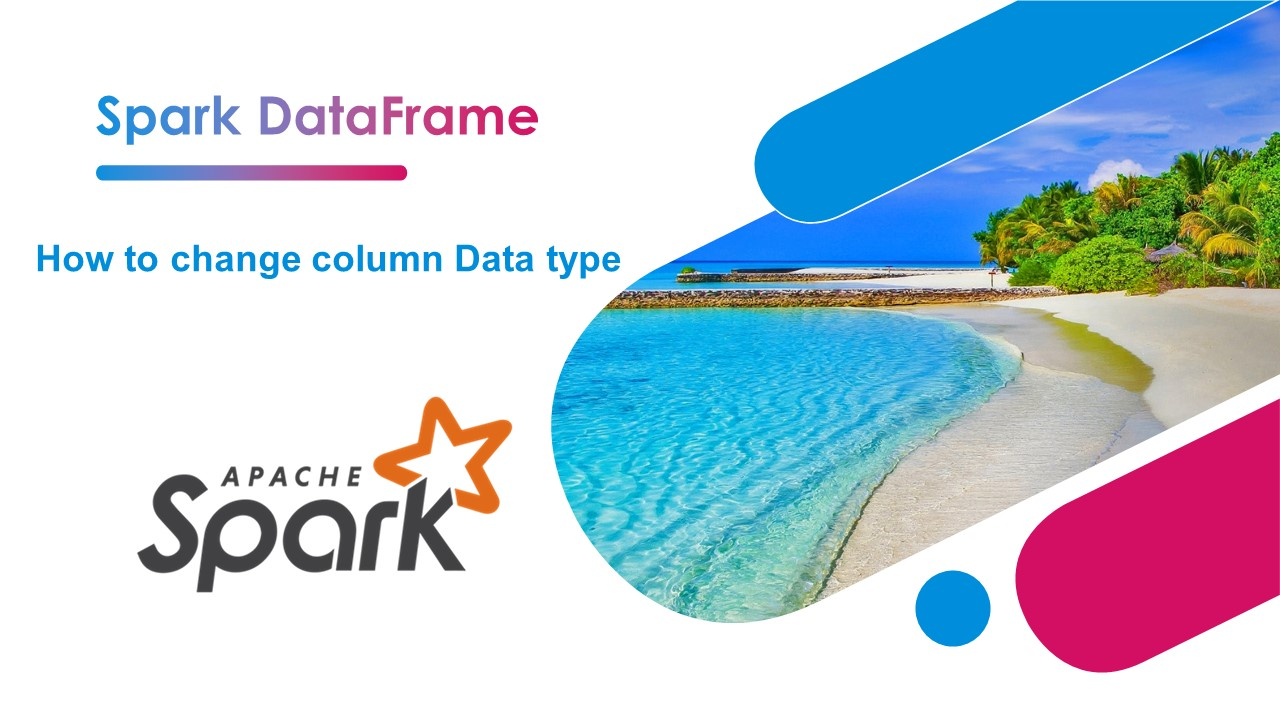# Spark – How to Change Column Type?

To change the Spark SQL DataFrame column type from one data type to another data type you should use `cast()` function of Column class, you can use this on withColumn(), select(), selectExpr(), and SQL expression. Note that the type which you want to convert to should be a subclass of DataType class or a string representing the type.

Key points:

• `cast()``<a href="https://sparkbyexamples.com/pyspark/pyspark-cast-column-type/">cast()</a>` is a function from `Column` class that is used to convert the column into the other datatype.
• This function takes the argument string representing the type you wanted to convert or any type that is a subclass of DataType (Below mentioned the types you could cast).
• When Spark unable to convert into a specific type, it returns a `null` value.
• Spark SQL takes the different syntax `DOUBLE(String column)` to cast types.
``````
import org.apache.spark.sql.functions.col
import org.apache.spark.sql.types.IntegerType

// Convert String to Integer Type
df.withColumn("salary",col("salary").cast(IntegerType))
df.withColumn("salary",col("salary").cast("int"))
df.withColumn("salary",col("salary").cast("integer"))

// Using select
df.select(col("salary").cast("int").as("salary"))

//Using selectExpr()

//Using with spark.sql()
spark.sql("SELECT cast(salary as int) salary, BOOLEAN(isGraduated),gender from CastExample")
``````

Let’s see some examples here using Scala snippet.

## Change Column Type Example

First, let’s create DataFrame.

``````
import org.apache.spark.sql.{Row, SparkSession}
import org.apache.spark.sql.types._
val spark: SparkSession = SparkSession.builder()
.master("local")
.appName("SparkByExamples.com")
.getOrCreate()

val simpleData = Seq(Row("James",34,"2006-01-01","true","M",3000.60),
Row("Michael",33,"1980-01-10","true","F",3300.80),
Row("Robert",37,"06-01-1992","false","M",5000.50)
)

val simpleSchema = StructType(Array(
StructField("firstName",StringType,true),
StructField("age",IntegerType,true),
StructField("jobStartDate",StringType,true),
StructField("gender", StringType, true),
StructField("salary", DoubleType, true)
))

val df = spark.createDataFrame(
spark.sparkContext.parallelize(simpleData),simpleSchema)
df.printSchema()
df.show(false)
``````

Outputs:

``````
root
|-- firstName: string (nullable = true)
|-- age: integer (nullable = true)
|-- jobStartDate: string (nullable = true)
|-- isGraduated: string (nullable = true)
|-- gender: string (nullable = true)
|-- salary: double (nullable = true)

+---------+---+------------+-----------+------+------+
+---------+---+------------+-----------+------+------+
|James    |34 |2006-01-01  |true       |M     |3000.6|
|Michael  |33 |1980-01-10  |true       |F     |3300.8|
|Robert   |37 |06-01-1992  |false      |M     |5000.5|
+---------+---+------------+-----------+------+------+
``````

## 1. Change Column Type using withColumn() and cast()

To convert the data type of a DataFrame column, Use withColumn() with the original column name as a first argument and for the second argument apply the casting method `cast()` with DataType on the column.

Below Spark, snippet changes DataFrame column, age to String (StringType), isGraduated column from String to Boolean (BooleanType) and ‘jobStartDate‘ column from String to DateType.

``````
import org.apache.spark.sql.functions._
val df2 = df.withColumn("age",col("age").cast(StringType))
.withColumn("jobStartDate",col("jobStartDate").cast(DateType))
df2.printSchema()
``````

Outputs:

``````
root
|-- age: string (nullable = true)
|-- isGraduated: boolean (nullable = true)
|-- jobStartDate: date (nullable = true)
``````

## 3. Using select() to Change Data Type

Below example cast’s selected columns using select() transformation. When you have many columns on DataFrame and wanted to cast selected columns this comes in handy.

``````
val cast_df = df.select(df.columns.map {
case [email protected]"age" =>
col(column).cast("String").as(column)
case [email protected]"salary" =>
col(column).cast("String").as(column)
case column =>
col(column)
}: _*)

cast_df.printSchema()
``````

`col(column).cast("String").as(column))` will be converted as `CAST(YEAR AS STRING) AS YEAR`. Yields below output.

``````
root
|-- firstName: string (nullable = true)
|-- age: string (nullable = true)
|-- jobStartDate: string (nullable = true)
|-- isGraduated: string (nullable = true)
|-- gender: string (nullable = true)
|-- salary: string (nullable = true)
``````

This example has been shared by @sriramrimmalapudi9gmail-com

## 3. Using selectExpr() to Change Data Type

Let’s use `selectExpr()` to convert spark DataFrame column `age` back to an integer, `isGraduated` from boolean to string and `jobStartDate` from date to String.

``````
val df3 = df2.selectExpr("cast(age as int) age",
"cast(jobStartDate as string) jobStartDate")
df3.printSchema()
df3.show(false)
``````
``````
root
|-- age: integer (nullable = true)
|-- isGraduated: string (nullable = true)
|-- jobStartDate: string (nullable = true)
``````

## 4. Using SQL Expression to Convert

We can also use SQL expression to change the spark DataFrame column type.

``````
df3.createOrReplaceTempView("CastExample")
DATE(jobStartDate) from CastExample")
df4.printSchema()
df4.show(false)
``````

Outputs:

``````
root
|-- age: string (nullable = true)
|-- isGraduated: boolean (nullable = true)
|-- jobStartDate: date (nullable = true)
``````

## 5. Complete Example of Casting DataFrame Column

``````
package com.sparkbyexamples.spark.dataframe

import org.apache.spark.sql.{Row, SparkSession}
import org.apache.spark.sql.types._
import org.apache.spark.sql.functions._

object CastColumnType extends App{
val spark: SparkSession = SparkSession.builder()
.master("local")
.appName("SparkByExamples.com")
.getOrCreate()

val simpleData = Seq(Row("James",34,"2006-01-01","true","M",3000.60),
Row("Michael",33,"1980-01-10","true","F",3300.80),
Row("Robert",37,"06-01-1992","false","M",5000.50)
)

val simpleSchema = StructType(Array(
StructField("firstName",StringType,true),
StructField("age",IntegerType,true),
StructField("jobStartDate",StringType,true),
StructField("gender", StringType, true),
StructField("salary", DoubleType, true)
))

val df = spark.createDataFrame(
spark.sparkContext.parallelize(simpleData),simpleSchema)
df.printSchema()
df.show(false)

val df2 = df.withColumn("age",col("age").cast(StringType))
.withColumn("jobStartDate",col("jobStartDate").cast(DateType))
df2.printSchema()

val df3 = df2.selectExpr("cast(age as int) age",
"cast(jobStartDate as string) jobStartDate")
df3.printSchema()
df3.show(false)

df3.createOrReplaceTempView("CastExample")
val df4 = spark.sql("SELECT STRING(age),BOOLEAN(isGraduated), " +
"DATE(jobStartDate) from CastExample")
df4.printSchema()
df4.show(false)

val cast_df = df.select(df.columns.map {
case [email protected]"age" =>
col(column).cast("String").as(column)
case [email protected]"salary" =>
col(column).cast("String").as(column)
case column =>
col(column)
}: _*)

cast_df.printSchema()
}
``````

This example is also available at GitHub for reference.

Happy Learning !!

### NNK

SparkByExamples.com is a Big Data and Spark examples community page, all examples are simple and easy to understand and well tested in our development environment Read more ..

### This Post Has 8 Comments

1.Naveen

I used Column() and cast(), works fine when used at individual files, but when tried same code for more than one file, it failed, as the schema for other files was most probably different, how to generalize the data type?

2.Anonymous

I used cast(IntegerType) but get this error: org.apache.spark.sql.AnalysisException: Cannot up cast from bigint to int.

3.Navin

I have use .selectExpr, it worked however this omits my other column in DF.

1.NNK

You should add all required columns into selectExpr(), This function results in a new DataFrame with just columns mentioned in it.

4.Anonymous

It always shows like name ‘NumericType’ is not defined, I don’t what I just missed from your article? I am so confused

1.NNK

Have you tried running complete example mentioned at the end of the post? just FYI, I’ve copied the code and tried it now and got the expected issue without any error. If you are still facing an error, please provide with a complete error stack trace.

2.You have not imported types – import org.apache.spark.sql.types._

1.NNK

Thanks Emil. I will add it.